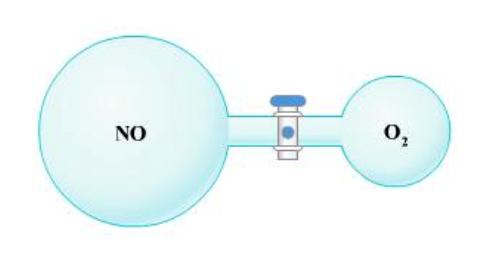# Problem: The picture below shows two bulbs connected by a stopcock. The large bulb, with a volume of 6.00 L, contains nitric oxide at a pressure of 0.850 atm, and the small bulb, with a volume of 1.50 L, contains oxygen at a pressure of 2.50 atm. The temperature at the beginning and the end of the experiment is 22°C. After the stopcock is opened, the gases mix and react: 2NO (g) + O2 (g) → 2NO2 (g) Which gases are present at the end of the experiment? What are the partial pressures in atm of the gases? If the gas was consumed completely, put 0 for the answer.

🤓 Based on our data, we think this question is relevant for Professor Rosensweig & Weiss' class at NU.

###### FREE Expert Solution###### Problem Details

The picture below shows two bulbs connected by a stopcock. The large bulb, with a volume of 6.00 L, contains nitric oxide at a pressure of 0.850 atm, and the small bulb, with a volume of 1.50 L, contains oxygen at a pressure of 2.50 atm. The temperature at the beginning and the end of the experiment is 22°C. After the stopcock is opened, the gases mix and react:

2NO (g) + O(g) → 2NO(g)

Which gases are present at the end of the experiment?

What are the partial pressures in atm of the gases? If the gas was consumed completely, put 0 for the answer.What scientific concept do you need to know in order to solve this problem?

Our tutors have indicated that to solve this problem you will need to apply the Partial Pressure concept. You can view video lessons to learn Partial Pressure. Or if you need more Partial Pressure practice, you can also practice Partial Pressure practice problems.

What is the difficulty of this problem?

Our tutors rated the difficulty ofThe picture below shows two bulbs connected by a stopcock. T...as high difficulty.

How long does this problem take to solve?

Our expert Chemistry tutor, Dasha took 16 minutes and 38 seconds to solve this problem. You can follow their steps in the video explanation above.

What professor is this problem relevant for?

Based on our data, we think this problem is relevant for Professor Rosensweig & Weiss' class at NU.